# The Programmatic Side of Mathematica V: Segue into Primes

You might recall in the last post the expansion of a general binomial$(a + b)^z$:

binexpand[z_] := Expand[(a + b)^z]
TableForm[Array[binexpand,6]]

You can also declare a loop in the way Mathematica does so that it gives a series of binomial expansions. Below is the output of the second statement from above, the first 6 expansions of a general binomial:

And if you want to mess around with the Map command, you can put square roots on everything:

You don’t always need to use Array[] to generate a list. You can also use Range[hi] which generates a list from 1 to “hi”:

Prime[Range]

Generates the first 100 primes:

{2, 3, 5, 7, 11, 13, 17, 19, 23, 29, 31, 37, 41, 43, 47, 53, 59, 61, 67, 71, \
73, 79, 83, 89, 97, 101, 103, 107, 109, 113, 127, 131, 137, 139, 149, 151, \
157, 163, 167, 173, 179, 181, 191, 193, 197, 199, 211, 223, 227, 229, 233, \
239, 241, 251, 257, 263, 269, 271, 277, 281, 283, 293, 307, 311, 313, 317, \
331, 337, 347, 349, 353, 359, 367, 373, 379, 383, 389, 397, 401, 409, 419, \
421, 431, 433, 439, 443, 449, 457, 461, 463, 467, 479, 487, 491, 499, 503, \
509, 521, 523, 541}

In earlier posts I had been curious about the distribution of prime numbers along the number line, and took my research to very large numbers, but I fear not large enough since I don’t have a supercomputer handy. My considered opinon first-hand, is that the primes are initially densely distributed, then gets gradually sparser in a way that is hard to predict. Mathematica claims it can easily access primes as high as on the order of$10^8$ in magnitude, and likely does the higher primes more slowly due to the fact that it uses primes up to that magnitude to search for still higher primes. If a natural number n has no prime numbers as factors, then n itself is prime.

The Mersenne primes, those Mersenne primes which are still being sought after, numbers large enough to fill every page in a 200-page novel, are notoriously hard to prove indivisible, since I would fear that the primes up to$10^8$ are not good enough. You need higher primes still, to many more orders of magnitude. Knowing the previous Mersenne prime is unreliable, since who knows how many primes lay between the current prime being checked and the previous one? There are likely to be hundreds, or even thousands of undiscovered primes which lay between$2^{p_{n-2}} - 1$ and$2^{p_{n-1}} - 1$, assuming$2^{p_{n-2}}$ and$2^{p_{n-1}}$ are prime (p, the exponent, is prime as well). The method of last resort, as I understand it, is to try every number up to$\lfloor \sqrt{2^{p}} \rfloor$ past$10^8$. While trying primes below$10^8$ weeds out several numbers, I think would still take a very long time for certain numbers that remain unfactored past that, possibly days to months even on a fast computer processor — to weed out one number or to successfully prove it as prime.

All other prime numbers which lay between two consecutive Mersenne primes (especially the big numbers) are nearly impossible to find, and I am not aware of anyone who bothers trying to find them (that doesn’t mean no one is trying, though). To find the next prime up from a Mersenne prime means looking to the next consecutive odd number, and the next, and the next, until you find one. A lot of time — months of time — is possibly wasted on a lot of composite numbers. Mersenne numbers narrow the search down, but only to a certain kind of prime number.

Mathematica has, for what it’s worth, a function called Prime[n], which returns the nth prime. Prime returns 2; Prime returns 3, and so on. The 100th prime is 541:

Prime
541

And if we subtracted

Prime - Prime
539

we can say that 539 integers lay between the first and the 100th prime. We can now do this:

Prime - Prime
1606

which tells us there is a difference of 1606 between the millionth prime and the 1,000,100th prime.

Prime - Prime
1974

The air gets thinner, but only slightly, when counting between the billionth prime and the 1,000,000,100th prime. By the trillionth prime, we get a difference of 3546 by the 1,000,000,000,100th prime. Or you can say 100 primes per 3546 consecutive integers. The trillionth prime is 29,996,224,275,833, or just under 30 trillion. Mathematica’s Prime[] function chokes when getting to numbers on the order of a quadrillion, even though the documentation claims arguments to Prime[] are allowed to go into the quintillions: 1,152,921,504,606,846,976 or 260.

I could say that this still provides an incredible list of primes for the possible writing of a prime factorization function, however, Mathematica has beat me to it, wtih the function FactorInteger[]. Even the Mathematica website at Wolfram Math World has defined a function that modifies the output of this function further:

FactorForm[n_?NumberQ,fac_:Automatic]:=
Times@@(HoldForm[Power[##]]&@@@FactorInteger[n,fac])

So, a call to FactorForm[] can be:

I don’t know what “Times@@” does, but I don’t seem to get anything other than the default terminal font. At any rate, the ordered pairs returned by FactorInteger[] are transformed into base integers and exponents.

# The Mersenne Prime Search

I have recently joined GIMPS, and have installed their prime search software on two of my computers.

Their website is at: mersenne.org.

# The Programmatic Side of Mathematica II

Since the last time I posted, I found a better way to do 100 dice rolls: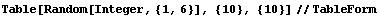This gives 100 dice rolls in a tabulated format in a much simpler syntax. You can also make a “die” out of complex numbers, but it is a little more of an issue to truncate: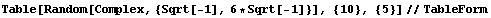… which gives output like: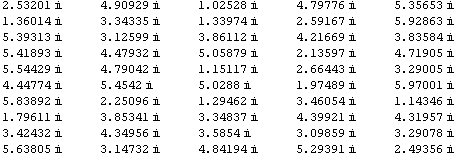Strictly speaking, these are all a kind of complex number called a pure imaginary number, but I guess I can’t split hairs.

You can create a list of divisors of integers like 28, like so:

Divisors
{1, 2, 4, 7, 14, 28}

28 is a called a perfect number, because like 6, the number can be created by the sum of its proper factors. A “proper factor” of a number k does not include k itself, so if you add the numbers generated in the above list, you will obtain 2k, or 56.

So to get the correct result, you can make your own function that can re-generate the list with the last element truncated. The Drop[] function can do this: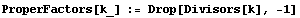I have declared a small function called ProperFactors, and the underscore after the k indicates that it is a placeholder for a parameter. When you run this, it returns nothing, but of course all you really did was declare a function. The Drop[] function drops elements from the list generated by the Divisors[] function, beginning at the last element (the reason there is a “-1” passed to Drop[]. In the list generated before, the last element was the highest factor of 28, which was 28 itself. The remaining elements in the list are the proper factors.

So, the call:

ProperFactors

generates the list:

{1, 2, 4, 7, 14}

which are the proper factors of 28. Let’s programmatically check to see if the sum of the proper factors add to 28. Of course, checking by adding the numbers up yourself will tell you this: 1 + 2 + 4 + 7 + 14 = 28. But making a function do the work will be beneficial if the factors become very large. So, we can make use of the Apply[] function:

Apply[Plus, {1,2,3,4,5}]

This returns:

15

or the sum of 1 + 2 + 3 + 4  + 5. It takes a list of numbers (an actual array), and treats them as though each element were parameters passed into the Plus[] function. I could get the same thing with:

Plus[1, 2, 3, 4, 5]
15

The curly braces aren’t needed, since Plus[] expects a list of arbitrary length, but not an array. Since ProperFactors[] generates an array, I need to convert the array into a list to be operated on by Plus[]. This is the reason for the Apply[] function.

List[] is a function which generates an array:

List[1,2,3,4,5]
{1, 2, 3, 4, 5}

And simply invoking the literal array {1, 2, 3, 4, 5} does the same thing as List[]. And you can assign the array to a variable.

s := List[2, 3, 4, 5, 6]

does the same thing as

s:= {2, 3, 4, 5, 6}

so that

Apply[Plus, s]

converts the array s to a list of parameters passed to Plus. The result is:

20

Now, let’s go back to our perfect number checking function. Let’s call it PerfectCheck[]:

PerfectCheck[k_] := Apply[Plus, ProperFactors[k]] == k

What does “Apply[Plus, ProperFactors[k]]” do? Let’s invoke that by itself in Mathematica to see, using k = 28:

Apply[Plus, ProperFactors]
28

so, it adds up the proper factors of 28. To make sure, let’s use a non-perfect composite number:

Apply[Plus, ProperFactors]
117

To check this, go further back and list the proper factors of 100 themselves: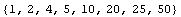You will see when adding by hand that these add to 117.

Thus, a PerfectCheck would be false since 117 <> 100.

PerfectCheck
false
PerfectCheck
true
PerfectCheck
true
PerfectCheck
false

Checking numbers in the range 1 to n can be done using Select[]:

perfect[n_] := Select[Range[n], PerfectCheck]

So that:

perfect

yields:

{6, 28, 496, 8128}

I was trying to find a fifth perfect number, but it’s difficult. I even cranked up the call to perfect but no luck, with Mathematica chugging away for about 10 minutes. But I was able to find information through Google more quickly. According to The Math Forum, they say that the fifth one was still some ways off: 33,550,336.

A sixth number may exist, but as you can see the trend seems to indicate that they are pretty sparse on the number line. The Math forum hinted that these perfect numbers obey a number pattern according to the formula: (2n)(2n+1 – 1). This isn’t really reliable; that is to say that the value of integer used for n will not generate the nth perfect number necessarily. This seems to generate a perfect number so long as 2n+1 – 1 equals the value of a Mersenne Prime. A Mersenne Prime is a prime number that is one less than some power of 2, such as the number 7, which is 23 – 1. When finding the “highest known prime number” these days, mathematicians look for Mersenne Primes.

Using a command like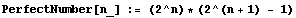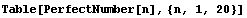can fool you into thinking you have just printed out the first 20 perfect numbers, but you would be wrong. The list it gives out: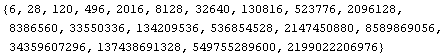has numbers, such as 120, 496, and 2016, that are not perfect. You still need to check each one with our PerfectCheck[] function (either that, or fish out the numbers with Mersenne prime factors among the lot).

You would have to keep hunting until you find 8,589,869,056 as being the sixth perfect number, closer to the end of the list. Then the seventh one is two more over: 137,438,691,328.

But, of course we have a computer, so life gets happy once again for the slack among us. We can mash up the Table[] call above, and Select[] numbers that are perfect with PerfectCheck: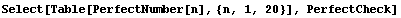which generates the array: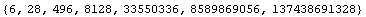and all of these are perfect numbers. I can go even more nutzo and increase the upper limit to 100: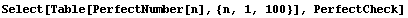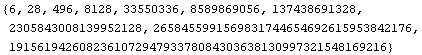… thereby generating the first 10 perfect numbers.

There are to date only 47 known Mersenne primes according to Wikipedia. Therefore, there are only 47  known perfect numbers, assuming all perfect numbers are even.

# More on primes

Yes, I’m still obsessed with prime numbers. I wrote a C program this time that can list or count prime numbers in a certain range, or do both. The C program is pretty fast, but in terms of large numbers, it takes a while to get the primes for large numbers.

I use something related to Euclid’s Algorithm for finding factors, except I turn things inside-out so that it avoids factors and finds primes. The program is quite short, about 60 or so lines, not counting comments. It was  a great way for me to freshen up my dusty C programming skills (I am recently more into Perl than anything).

The hardest part of writing this program seemed to be in getting it to accept numbers from the command line. This was because I needed to review all that about strings, pointers, casting, string conversion, and how argv[] and argc are structured.

Once it was written, however, I could use it to find primes in numbers that were nearly as big as I wanted. It couldn’t take scientific notation, which would be a bit more work to parse from the command line. I was happy with just using raw integers, even though many of them were on the order of 1012 and beyond.

One of the great advantages of having a small C program is that I could log on to several different UNIX machines (Linux mostly), and run an instance of the program on a different range of numbers. This was very helpful when the numbers were in the trillions, because I was aware I was on a timesharing system on each machine, and that looking for primes would push up the load average quite noticeably for lengths of time extending for as much as 3 hours, and having several processes on the same machine used in tandem by other users just wouldn’t do.

I used  the UNIX time command to find out how long my program takes to find primes in real time. It was intended for my own information, but I wished I had recorded the data, since if I ever find out about an improved algorithm, or make improvements myself, it would be nice to have times I can compare these to.

The largest numbers input were on the order of 1019, a number so big I needed to significantly reduce the range of numbers it searches in to 1000. To make a search of only 1000 integers, the program still took about 20 minutes for a range such as 5⋅1019 to 5⋅1019 + 1000. I found this law of diminishing returns quite unsatisfying. The higher the number the shorter the range you had to give it, unless you wanted the program to take forever.

# In Pursuit of Trends in Primes

INTRODUCTION

The prime numbers are numbers which are divisible only by itself and 1. This means that all primes have two factors, so for that reason, “1” is not prime.

OBSERVATIONS

The first 100000 integers seems to have the greatest density of prime numbers. 9592 primes were found there, meaning on average, nearly one in 10 of the first 100000 integers were prime.

Dissecting this interval further, for the first 1000 contiguous integers there are 168 primes:

 INTERVAL    # OF PRIMES  FRACTION
1-100          25        0.25
101-200          21        0.21
201-300          16        0.16
301-400          16        0.16
401-500          17        0.17
501-600          14        0.14
601-700          16        0.16
701-800          14        0.14
801-900          15        0.15
901-1000         14        0.14
~~~~~~~~~~~~~~~~~~~~~~~~~~~~~~~~~~
TOTAL           168        0.168

we see we’re already in trouble. While the first 200 integers clearly show dominance in having the most primes, with the first 100 integers having the most of all, the remainder fall into some kind of pseudo-random torpor, with some intervals higher, and some lower. 16 primes occur in three of the intervals, and 14 occur in another three.

In this interval, it is difficult to figure out if the numbers are meandering up or down. Let’s look at the second thousand:

1001-1100    16    0.16
1101-1200    12    0.12
1201-1300    15    0.15
1301-1400    11    0.11
1401-1500    17    0.17
1501-1600    12    0.12
1601-1700    15    0.15
1701-1800    12    0.12
1801-1900    12    0.12
1901-2000    13    0.13
~~~~~~~~~~~~~~~~~~~~~~~~~~~~~~~~~~
TOTAL:      135    0.135

Is this a downward trend? We observe the first 10 thoudsands:

   1-1000    168    0.168
1001-2000    135    0.135
2001-3000    127    0.127
3001-4000    120    0.120
4001-5000    119    0.119
5001-6000    114    0.114
6001-7000    117    0.117
7001-8000    107    0.107
8001-9000    100    0.100
9001-10000   112    0.112
~~~~~~~~~~~~~~~~~~~~~~~~~~~~~~~~~~~
TOTAL:      1219    0.1219

A major jump upward is observed in the last interval, but it does not get to the level of the first 4 intervals. This is noticed nearly universally, and is reproduced here. If you look at the number of primes in regularly-spaced intervals of contiguous sequences of integers with each interval the same size, you tend to see a general declining trend, followed by a seemingly random meanindering of numbers of primes going up and down in number. This observation appears to be independent of
interval size.

In the interval 99001 to 100000, the last 1000 numbers in the interval, we observe that there are 86 primes. Clearly, this is below what we have just observed in the first 10 intervals of 1000, but in the interval 97001-98000, we observe only 82 primes, where we had expected a slightly greater number than 86. The number of primes once again are returning to a similar pseudo-random torpor observed earlier.

I define “pseudo” randomness as frequencies which go on a downward trend in an unpredictably meandering way. True randomness would have frequencies going all over the place.

So, if intervals of size “p” were used, the first p integers would contain the most primes; while there would be a downward trend for integers from p+1 to 2p; 2p+1 to 3p, and so on. By some middle interval kp+1 to (k+1)p, there would occur a relatively low number of primes in some pseudo random torpor. By the time we arrived at interval np+1 to (n+1)p, the frequency of primes become noticeably fewer. I am afraid I am not yet in a position to define the word “noticeably”. You just have to notice it.

But the word “noticeably” might imply that there is an upper limit of frequencies which it does not attain. That is, if “e” was the upper limit of a predicted frequency by interval np+1 to (n+1)p, we need to set the number so as to guarantee to ourselves that it does not rise above the limit. As a first approximation, I am willing to set e, if 2 divides n, as being the same for the interval (n/2)p+1 to ((n/2)+1)p, or the interval half-way to the last one. You obviously can be more restrictive than that, but at least you can see from my numbers that this clearly works out.

Contiguous Intervals of 100000

Taking the whole interval of the first 100000 positive integers contiguously, we find there are 9592 primes, far and away more than any other such interval observed for 100000 integers. The second interval from 100001 to 200000, has 8392 primes. Going from 1 billion, the interval has 4832 primes. While it is not clear if we are returning to torpor, we can see at least that the number of primes are decreasing.

The strongest proof I have observed of a decline returning to a chaos of up-and-down numbers of primes, was to see that these observations were even consistent when I counted 100,000 contiguous integers, and jumping 10^50 integers and counting another interval of 100,000 from there. I kept this up under Maple 9.5 up to 10^2000. The last intervals from 10^1700 took the longest to count (well, _you_ try checking 100000 integers which are each 1700 digits long, and see how long it takes you!). It is still cranking away, and there are 4 intervals left. In all, it will probably take 9 hours on a dual-core processor, so on the optimistic side, I have about 3 hours remaining.

The results so far have been enough to more than indicating a trend:

100000 FROM   # OF PRIMES     EQUAL OR GREATER(*)
10^0            9592
10^50            895
10^100           407
10^150           274
10^200           216
10^250           165
10^300           143
10^350           129
10^400           115
10^450            96
10^500            81
10^550            71
10^600            78                   *
10^650            65
10^700            68                   *
10^750            59
10^800            52
10^850            57                   *
10^900            57                   *
10^950            45
10^1000           39
10^1050           38
10^1100           43                   *
10^1150           33
10^1200           38                   *
10^1250           43                   *
10^1300           21
10^1350           32                   *
10^1400           28
10^1450           28                   *
10^1500           26
10^1550           35                   *
10^1600           32
10^1650           28
10^1700           28                   *
10^1750           29                   *
10^1800           27
10^1850           23

As has been stated by other writers, prime numbers appear to have a pattern, but the pattern has eluded us. It seems the pattern of decreasing numbers of primes as integers increase by many orders of magnitude seems to be elusive indeed, appearing pseudo-random, but with a general downward trend. One might desire to regress the numbers of primes per 100,000 integers to a curve, but I am not sure that would tell us anything meaningful by way of a pattern.